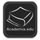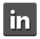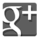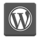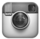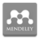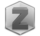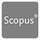You are in:Home/Theses

### Dr. Mohamed Ahmed Kamal Abd-El-Khalik Ahmed Soliman :: Theses :

 Title Dynamic Analysis of Unbounded Domain Problems by applying the SBFEM Type MSc Supervisors Abd-Elrahman Ali Saad; Emad Hassan Zahran Year 2015 Abstract This Thesis presents an explanation of the scaled boundary finite element method (SBFEM) as a method to solve unbounded domain problems subjected to dynamic loads with application on dynamic soil structure interaction problems in time domain. A complete derivation of the SBFE equations for unit impulse response function M (t) is made; the equations are solved numerically assuming the unit impulse response function as piece wise linear taking the advantage that M (t) becomes nearly linear after a certain time , where the time step used in calculating the unit impulse is greater than that used in calculating the response, which reduces the time of analysis greatly. The response of the unbounded domain is calculated, where the force displacement relationship is calculated at each time step using integration by parts . Also coupling between the finite element method (FEM) and the SBFEM is used. A program made by the author is used to solve the problems using the SBFEM and the coupled FE/SBFE methods using MATLAB. Some benchmark problems are solved as a validation; also some practical problems are solved to show the efficiency of the method, where the solution is checked using Finite element method. Good agreement is achieved between results in case of benchmark problems and the practical problems. So, the scaled boundary finite element method is an acceptable method in modelling soil structure interaction problems and also it saves a lot of time in comparison to finite element method. Keywords Scaled boundary Finite element method - Dynamic stiffness - unbounded domain University Benha Country Egypt Full Paper download paper

 Title Damage Mechanics Modeling Using Eshelby Inclusions Type PhD Supervisors Abd-Elrahman Ali Saad; Youssef F. Rashed; T. H. Naga; Ahmed Fady Farid Year 2021 Abstract This Thesis consists of three parts: In the first part of the thesis, the idea of the Eshelby equivalent inclusion theory is coupled with the Fictitious stress method (FSM) as a meshless technique to model inhomogeneity problems. The FSM is regarded herein as an indirect boundary element formulation. The problem is divided into complementary and particular parts. The particular solution is obtained using Eshelby theory. Hence the complementary solution could be obtained using the FSM. Analytical solutions are used to model circular inclusions. In this approach there is no meshing on the boundary or inside the domain. The problem is solved by distributing points on the boundary and inserting points at the center of the inhomogeneities. Although the fictitious stresses on the boundary is assumed constant and also the eigenstrain inside the inclusion is assumed uniform the results are in good agreement with the direct boundary integral equation method and the finite element method as will be shown in chapter 3. In the second part of the thesis, a new simulation of damage in the direct boundary element formulation is presented. The Eshelby equivalent inclusion theory is coupled with the direct boundary integral equation to model the change in the elastic properties due to damage. A finite element-like stiffness matrix is formed for the damaged domain, where the problem stiffness matrix is obtained directly on the boundary (in condensed form). The developed method is a boundary-only method although the domain contains damaged parts. A system of nonlinear equation is then solved using a load control approach (secant method). Both local and non-local damage models are considered. Several examples are presented to demonstrate the validity and the accuracy of the proposed formulation as will be shown in chapter 4. In the third part, the application of the damage modelling within the finite element method is reviewed. Hence the basic theory of the variational boundary integral equations (VBIE) is discussed, where a finite element-like stiffness matrix obtained using VBIE is used to model the damage. The VBIE gives the ability to use large dimensions compared to the conventional finite element. Although using coarse mesh the results as will be shown in chapter 5 are in good agreement with the conventional finite element. Keywords DAmage Mechanics; Eshelby theory; equivalent inclusion; nonlocal damage; Fictitious stress method; particular integral University Benha Country Egypt Full Paper download paper